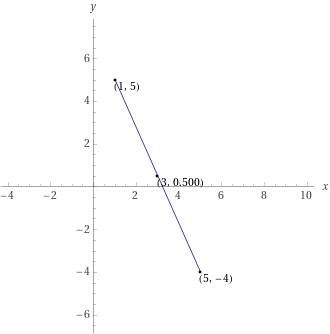Search IntMath
Close

450+ Math Lessons written by Math Professors and Teachers

5 Million+ Students Helped Each Year

1200+ Articles Written by Math Educators and Enthusiasts

Simplifying and Teaching Math for Over 23 Years

# Understanding Midpoint in Geometry## What is Midpoint?

Midpoint is an important concept in geometry, used to describe the halfway point between two points on a line, plane, or triangle. Midpoint is also known as a centroid, and is used to calculate the distance between two points. With midpoint, you can easily calculate the area of a triangle, the length of a line, or the perimeter of a plane.

Midpoint is derived from the Greek words "mid" and "point," literally meaning the middle point. It is a common geometrical concept in mathematics, used to measure distances, angles, and other properties of shapes. Midpoint is also used to determine the center of a circle, the center of a triangle, or the center of a polygon.

## Geometric Midpoint

A geometric midpoint is the point that lies halfway between two other points on a line, plane, or triangle. It is usually represented by a dot on a graph, and is used to calculate the distance between two points. The midpoint of a line is the point that divides the line into two equal parts. The midpoint of a triangle is the point at which all three sides of the triangle meet.

In order to calculate the midpoint of a line, the midpoint formula is used. The formula for the midpoint of a line is: M=(x1+x2)/2, where x1 and x2 are the x-coordinates of the two points on the line. The y-coordinate of the midpoint is calculated in a similar way: M=(y1+y2)/2.

## Midpoint Theorem

The midpoint theorem states that the midpoint of a line is the point at which the line is divided into two equal parts. The midpoint theorem can be used to calculate the length of a line, the area of a triangle, or the perimeter of a plane. The midpoint theorem is also used to calculate the center of a circle, the center of a triangle, or the center of a polygon.

The midpoint theorem can be used to calculate the length of a line segment by using the midpoint formula. The formula for the length of a line segment is: L=v((x1-x2)2 + (y1-y2)2), where x1 and x2 are the x-coordinates of the two points on the line and y1 and y2 are the y-coordinates of the two points on the line.

## Midpoint of a Triangle

The midpoint of a triangle is the point at which all three sides of the triangle meet. The midpoint of a triangle can be used to calculate the area of a triangle, the perimeter of a triangle, or the center of a triangle. The formula for the area of a triangle is: A=1/2ah, where a is the length of one side of the triangle and h is the height of the triangle. The formula for the perimeter of a triangle is: P=a+b+c, where a, b, and c are the lengths of the sides of the triangle. The formula for the center of a triangle is: C=(x1+x2+x3)/3, where x1, x2, and x3 are the x-coordinates of the three points of the triangle.

## Practice Problems

1. Find the midpoint of the line segment with endpoints (2,3) and (7,9)

2. Find the length of the line segment with endpoints (5,4) and (1,7)

3. Find the area of a triangle with sides of length 5, 6, and 7

4. Find the perimeter of a triangle with vertices (2,3), (7,9), and (4,7)

5. Find the center of a triangle with vertices (1,2), (3,4), and (5,6)

## Summary

Midpoint is an important concept in geometry, used to describe the halfway point between two points on a line, plane, or triangle. A geometric midpoint is the point that lies halfway between two other points on a line, plane, or triangle. The midpoint theorem states that the midpoint of a line is the point at which the line is divided into two equal parts. The midpoint of a triangle is the point at which all three sides of the triangle meet. The midpoint theorem can be used to calculate the length of a line, the area of a triangle, or the perimeter of a plane. The midpoint formula is used to calculate the midpoint of a line and the midpoint theorem can be used to calculate the length of a line segment, the area of a triangle, the perimeter of a triangle, or the center of a triangle.

## FAQ

### What is a midpoint?

In geometry, the midpoint is the point that is halfway between two other points on a line segment.

### How is a midpoint determined?

A midpoint is determined by taking the average of the x-coordinates and the average of the y-coordinates of the two points that make up the line segment.

### What is the formula for finding the midpoint?

The formula for finding the midpoint is as follows: Midpoint = (x1 + x2) / 2, (y1 + y2) / 2.

## Problem SolverThis tool combines the power of mathematical computation engine that excels at solving mathematical formulas with the power of GPT large language models to parse and generate natural language. This creates math problem solver thats more accurate than ChatGPT, more flexible than a calculator, and faster answers than a human tutor. Learn More.